Next: MINIMUM K-SATISFIABILITY Up: Propositional Logic Previous: MAXIMUM SATISFIABILITY   Index

### MAXIMUM K-SATISFIABILITY

• INSTANCE: Set U of variables, collection C of disjunctive clauses of at most k literals, where a literal is a variable or a negated variable in U. k is a constant,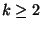.

• SOLUTION: A truth assignment for U.

• MEASURE: Number of clauses satisfied by the truth assignment.

• Good News: Approximable within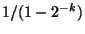if every clause consists of exactly k literals .

• Comment: MAXIMUM 3-SATISFIABILITY is approximable within 1.249  and is approximable within 8/7 for satisfiable instances . MAXIMUM K-SATISFIABILITY is not approximable withinfor anyand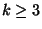, even if every clause consists of exactly k literals .

MAXIMUM 2-SATISFIABILITY is approximable within 1.0741 , and is not approximable within 1.0476 . The weighted version of this problem is as hard to approximate as the unweighted version . If every clause consists of exactly k literals, the weighted version of the problem is as hard to approximate as the unweighted version .

Variation in which the number of occurrences of each variable is bounded by a constant B for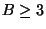is still APX-complete  and ; for B=6 it is not approximable within 1.0014 . If every clause consists of exactly three literals and the number of occurrences of each variable is exactly 3, then the problem is polynomially solvable (see Problem 9.5.4 of ), but for exactly five occurrences, the problem is APX-complete .

Admits a PTAS if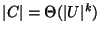. Variation in which each clause is a Horn clause, i.e., contains at most one nonnegated variable, is APX-complete, even for k=2 .

• Garey and Johnson: LO2 and LO5Next: MINIMUM K-SATISFIABILITY Up: Propositional Logic Previous: MAXIMUM SATISFIABILITY   Index
Viggo Kann
2000-03-20